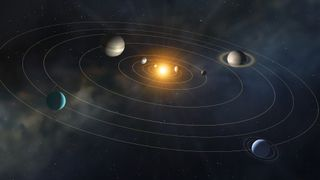# What makes Newton's laws work? Here's the simple trick.Paul M. Sutter is an astrophysicist at SUNY Stony Brook and the Flatiron Institute, host of "Ask a Spaceman" and "Space Radio," and author of "How to Die in Space."

We all learned Newton's laws in high school: Objects in motion tend to stay in motion, force equals mass times acceleration, and for every action, there is an equal and opposite reaction. From those laws of motion, Isaac Newton discovered a universal theory of gravity that applied equally well to apples falling from trees and planets moving in their orbits.

But Newton couldn't explain why his laws of motion were correct, and why they didn't have some other form. That discovery would come from another legendary, but less-famous genius.

## Lagrange vs. Newton

We're used to thinking of motion in terms of forces and accelerations — partly because that's a very intuitive way to view the world (for example, I push on something, and it moves) and partly because that's how Newton formulated his laws (and hence how we're taught them in school).

But examining forces and masses isn't the only way to describe the world around us. Think about a ball being thrown in the air. That ball has a lot of properties that we might find useful — things like its position, velocity, acceleration and mass. Some of these properties may be very useful in predicting the ball's future motion, and some less so.

Newton found that the combination of mass, acceleration and force was very powerful indeed, allowing him to formulate his famous Force = mass * acceleration equation as a fundamental law of the universe.

About 150 years after Newton developed his laws of motion, another mathematician, physicist and all-around genius, Joseph Louis Lagrange, developed his own formulations. He found that by looking at the kinetic and potential energies of an object, he could also derive his own laws of motion.

Specifically, Lagrange found that the difference between an object's kinetic energy and potential energy unlocked something deeply profound about the universe.

## Stationary action

If I were to throw a ball at you, you would probably have a decent chance of catching it. You can do this because in your lifetime, you've seen lots of balls thrown at you, and your brain has deciphered that thrown objects follow a rather common set of trajectories. Newton's insight was his ability to find a general law of motion that could predict the trajectory of that thrown ball.

But why should Newton's laws be correct? Why should a thrown ball follow the familiar path? Why don't balls jump backward first, or shoot off to Mars on their way to you? Why does the same path happen every single time? In other words, why do objects behave the way they do, rather than any other way? The universe could have chosen literally any behavior for thrown balls or any other objects in motion. What makes Newton's laws work?

Newton didn't have the answer, but Lagrange did.

The key is the difference between the kinetic and potential energies of the object in motion. If you watch a ball in flight, for example, then at every moment in time, you can calculate that difference. At the end of the motion, you can add up all those differences and get a single number. This number is called, for various historical reasons, the action of the object in motion.

You can imagine different possible paths the ball might take as it's thrown to you. These different possible paths will have different actions associated with them. And it turns out that the familiar path ​​— the path exactly predicted by Newton's laws — is the path with the least possible amount of action.

## Creating laws of motion

Lagrange discovered what today we call the principle of least action. All physical laws, including Newton's laws, flow from this single unifying principle.

To craft a law of motion, you follow a simple recipe. First, you write down the kinetic and potential energies of the objects of interest. Then, you take their difference. (We now call this quantity "the Lagrangian" in his honor.) Then, you apply a fancy mathematical technique called the calculus of variations to find the expression that minimizes the action. What pops out is a brand spankin' new law of physics.

All of modern physics is written in this language, because it's such a powerful and clever (and universal) way to approach dynamics. General relativity, electromagnetism, and even quantum field theory and the Standard Model all start off as Langrangians, and physicists around the world apply Lagrange's rules to derive laws of motion.

Those laws of motion include those that govern the movement of the planets in the solar system and the expansion of the universe itself. Whether you're using general relativity or the original Newtonian version of gravity, Lagrange's trick will always give you the answers you seek.